﻿ 复合式气囊隔振器垂向静态特性研究
 舰船科学技术2016, Vol. 38Issue (6): 97-100,104PDF

1. 海军工程大学 振动与噪声研究所, 湖北 武汉 430033 ;
2. 船舶振动噪声重点实验室, 湖北 武汉 430033

Investigation on vertical static properties of a complex air spring
DU Cong-ru1,2, ZHAO Ying-long1,2
1. Institute of Noise and Vibration, Naval University of Engineering, Wuhan 430033, China ;
2. National Key Laboratory on Ship Vibration & Noise, Wuhan 430033, China
Abstract: This paper investigates calculation method for vertical static properties of a complex air spring. The finite element analysis and experiment are conducted upon it. By comparison, the vertical static properties obtained by simulation and experiment test respectively are approximately consistent with the theoretical calculation results, which shows the method of theoretical calculation is effective. The method of theoretical calculation for vertical static properties provides a reference for other kinds of isolators.
Key words: complex air spring     vertical static properties     finite element analysis
0 引 言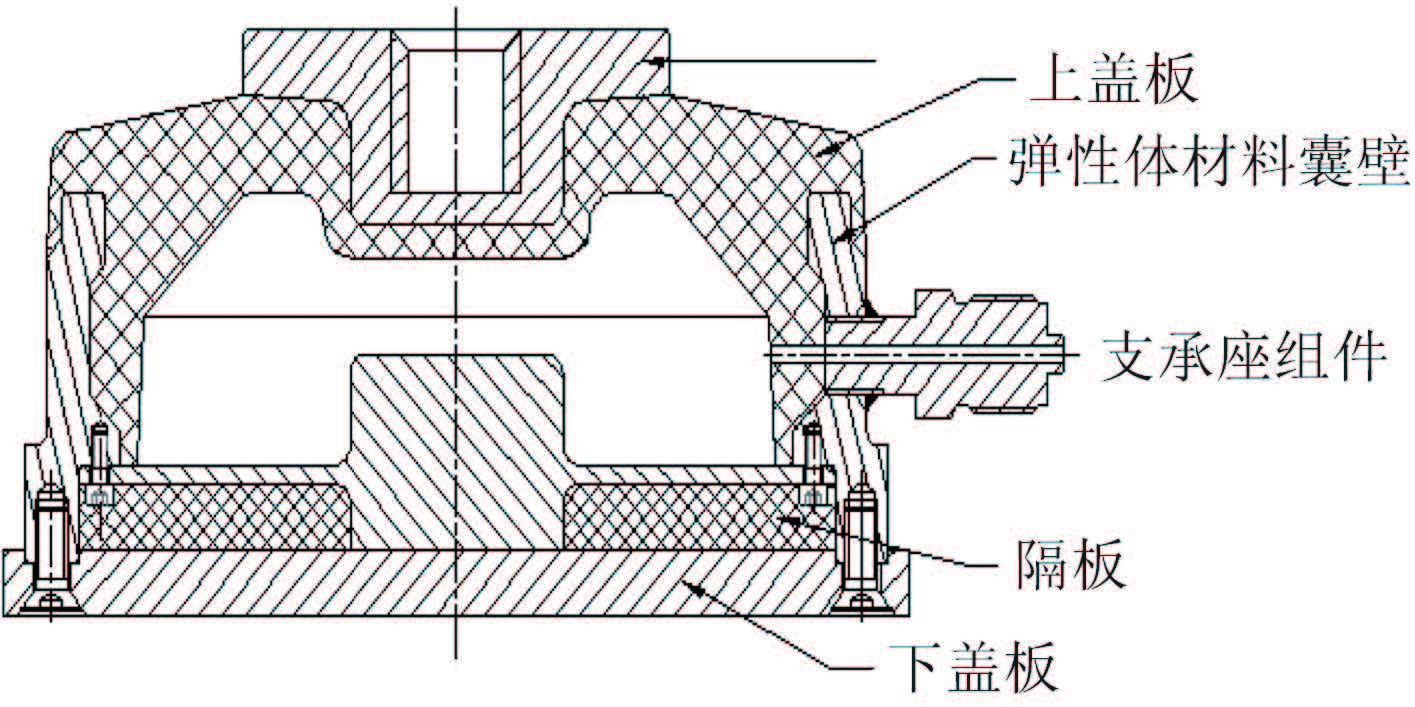图 1 复合式气囊隔振器结构图 Fig. 1 Schematic diagram of the complex air spring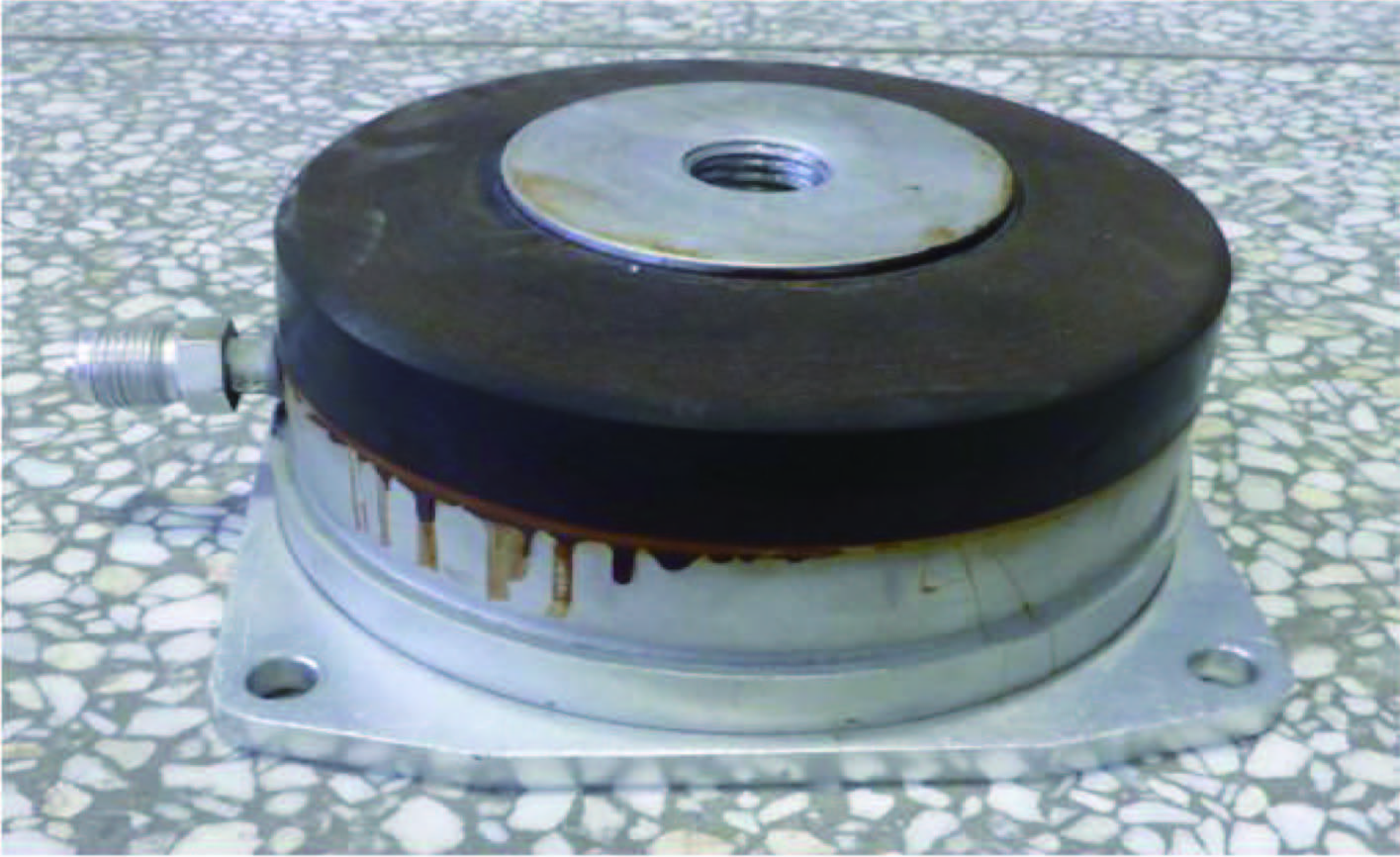图 2 复合式气囊隔振器实物图 Fig. 2 Complex air spring

1 垂向静态特性计算研究

 $k = {k_k} + {k_t}.$ (1)

1.1 弹性体囊壁的垂向静刚度计算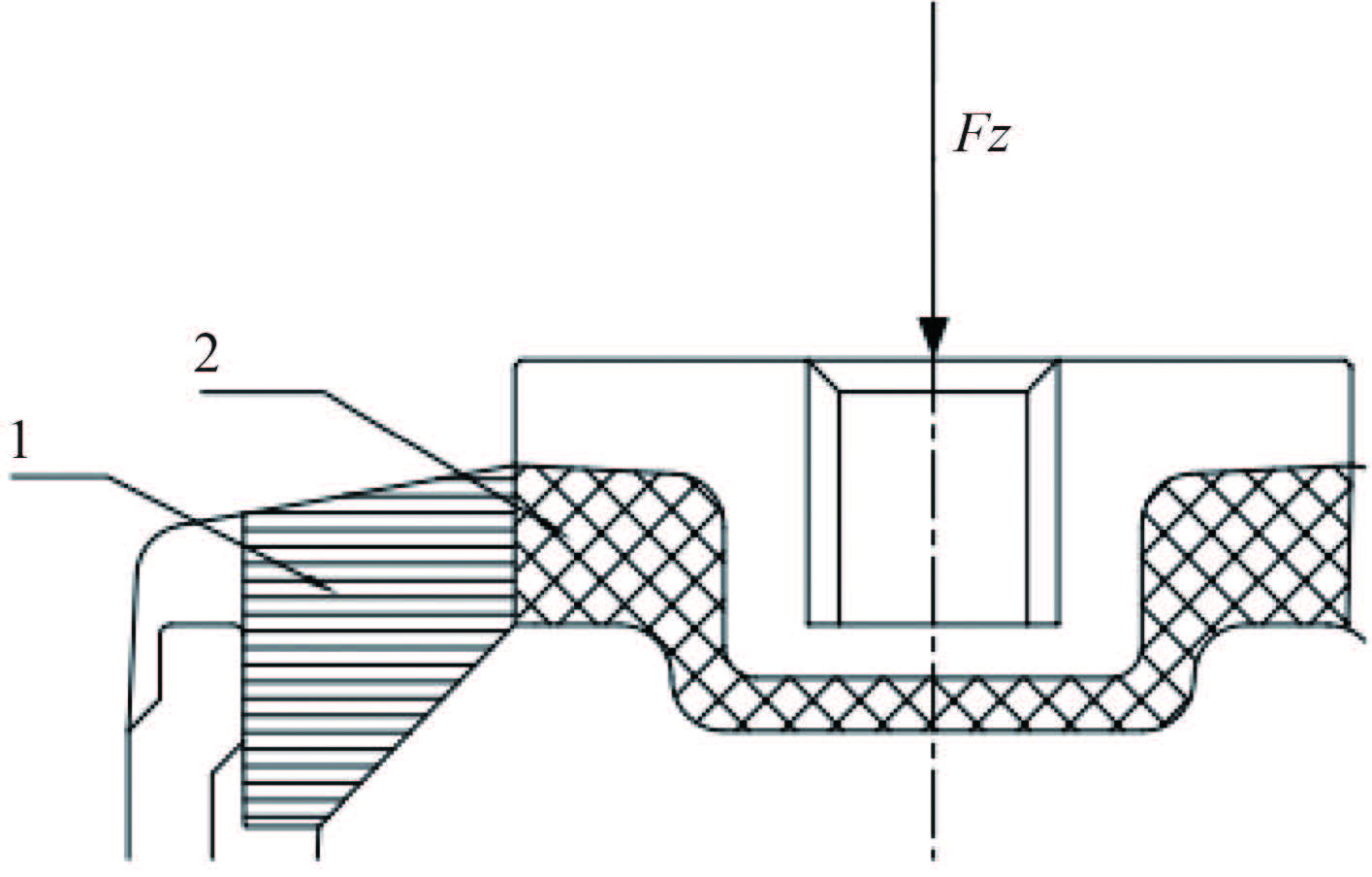图 3 隔振器局部图 Fig. 3 A part of complex air spring

 ${k_t} = \frac{{2{G_{ap}}({l_2}{r_1} - {l_1}{r_2})}}{{({r_2} - {r_1}){\rm{ln}}\frac{{{l_2}{r_1}}}{{{l_1}{r_2}}}}}.$ (2)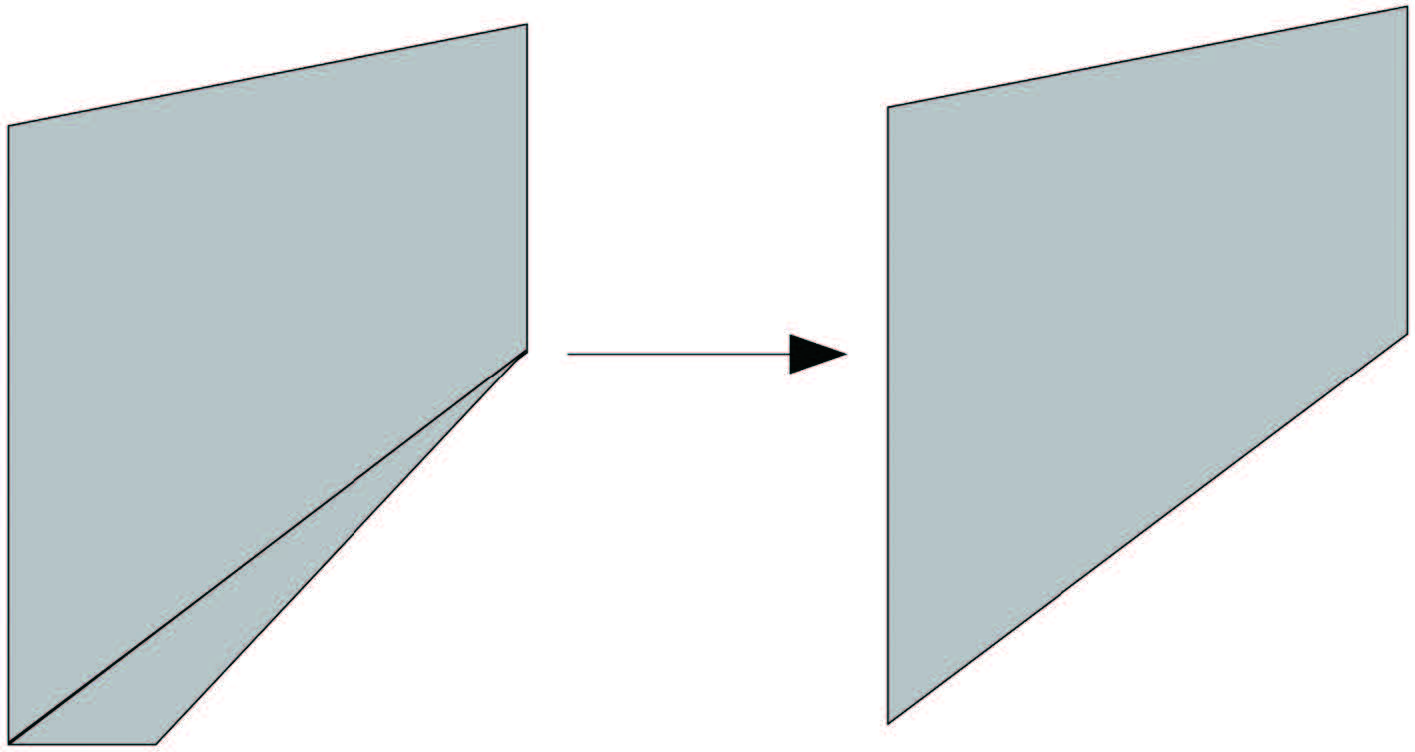图 4 弹性体受力部分简化图 Fig. 4 The simplified diagram of elastic body on load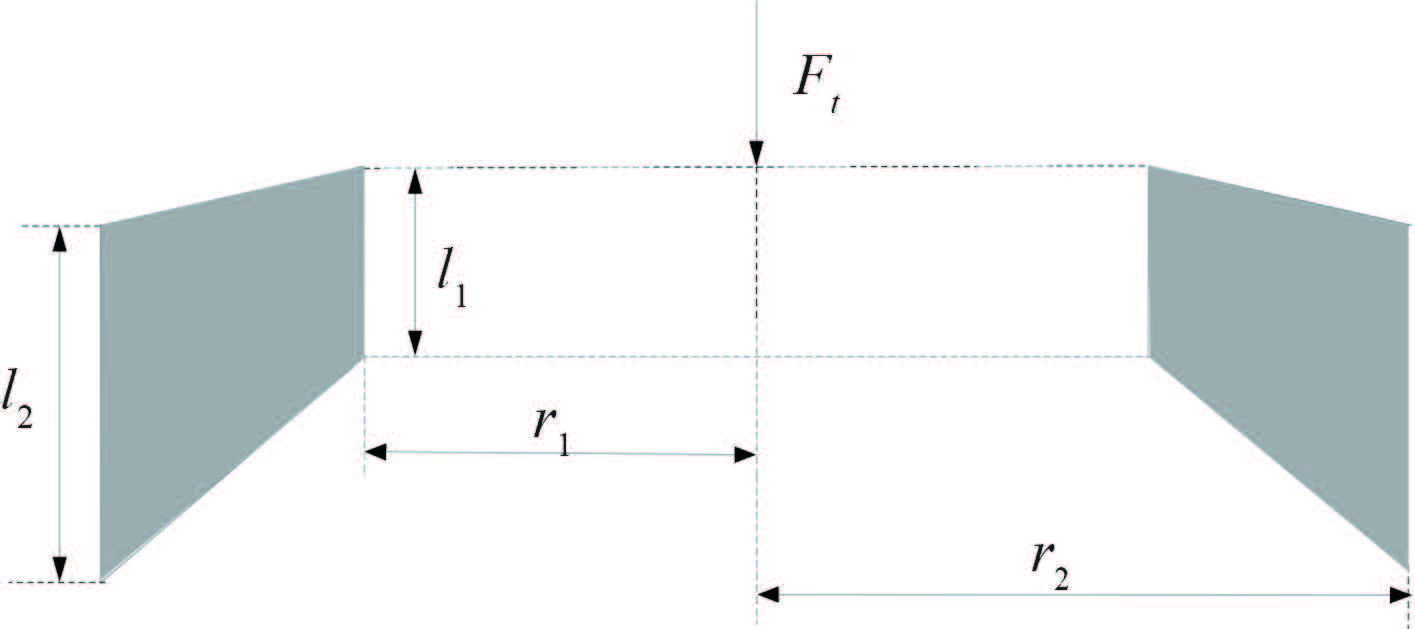图 5 弹性体计算模型 Fig. 5 Calculation model of elastic body

 ${G_{ap}} = m \times G.$ (3)

 $m = 1 + \frac{1}{3}\frac{{{{({r_2} - {r_1})}^2}}}{{{l_1}{l_2}}}.$ (4)
1.2 气囊的垂向静刚度计算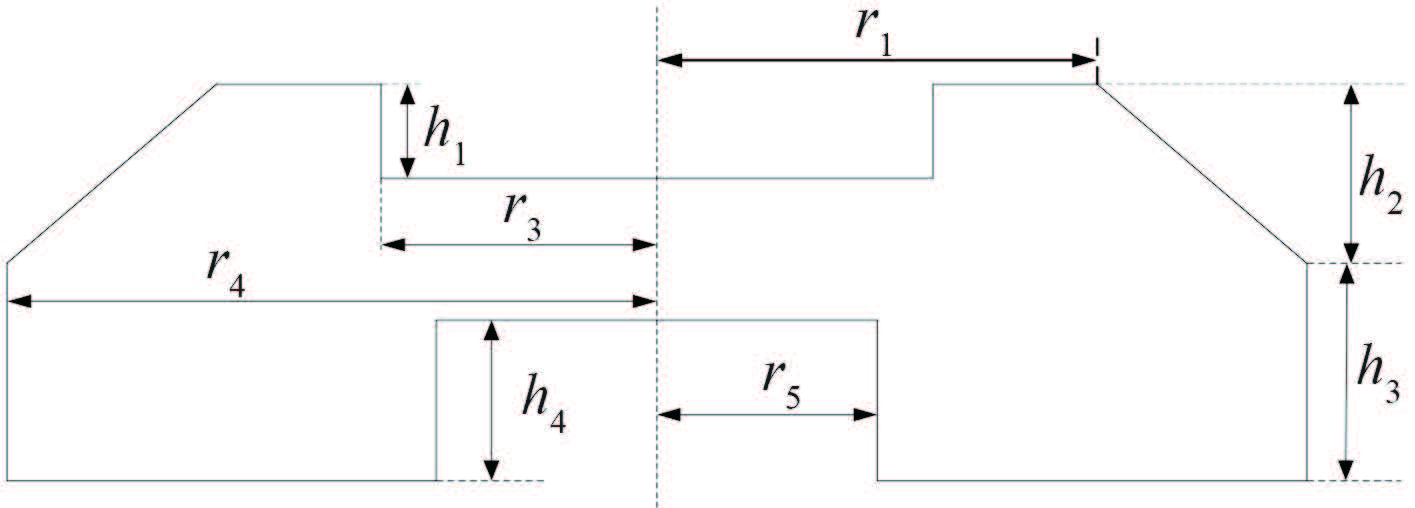图 6 气囊的计算模型 Fig. 6 Calculation model of air cavity

 ${F_k} = P{A_{eff}}.$ (5)

 ${F_k}dZ + P{\rm{d}}V = 0.$ (6)

 ${A_{eff}} = \frac{{{F_k}}}{P} = - \frac{{{\rm{d}}V}}{{{\rm{d}}Z}}{\rm{.}}$ (7)

 ${V_0} = \frac{\pi }{3}{h_2}(r_4^2 + r_1^2 + {r_1}{r_4}) - \pi r_3^2{h_1} + \pi r_4^2{h_3} - \pi r_5^2{h_4}.$ (8)

 $V = \frac{{\pi ({h_2} - Z)}}{3}(r_4^2 + r_1^2 + {r_1}{r_4}) - \pi r_3^2{h_1} + \pi r_4^2{h_3} - \pi r_5^2{h_4}.$ (9)

 ${A_{eff}} = \frac{\pi }{3}(r_4^2 + r_1^2 + {r_1}{r_4}).$ (10)

 $(P + {P_a}){V^n} = ({P_0} + {P_a})V_0^n{\rm{.}}$ (11)

 $P = ({P_0} + {P_a}){(\frac{{{V_0}}}{V})^n} - {P_a}{\rm{.}}$ (12)
 ${F_k} = P(Z){A_{eff}}(Z).$ (13)

 ${k_k} = \frac{{{\rm{d}}{F_k}}}{{{\rm{d}}Z}} = n\frac{{(P + {P_a})}}{V}{A_{eff}}^2{\rm{.}}$ (14)

1.3 隔振器的垂向静刚度

 $k = {k_t} = \frac{{2\pi {G_{ap}}({l_2}{r_1} - {l_1}{r_2})}}{{({r_2} - {r_1}){\rm{ln}}\frac{{{l_2}{r_1}}}{{{l_1}{r_2}}}}}.$ (15)

 $k = {k_t} = \frac{{2\pi {G_{ap}}({l_2}{r_1} - {l_1}{r_2})}}{{({r_2} - {r_1}){\rm{ln}}\frac{{{l_2}{r_1}}}{{{l_1}{r_2}}}}}{\rm{.}}$ (16)
1.4 实例计算表 1 复合式气囊隔振器结构尺寸 Tab.1 Structure dimension of complex air spring

2 仿真分析 2.1 隔振器聚氨酯弹性体材料特性

 $W = \sum\limits_{i = 1}^N {\frac{{{\mu _i}}}{{{\alpha _i}}}} \left( {\lambda _1^{{\alpha _i}} + \lambda _2^{{\alpha _i}} + \lambda _3^{{\alpha _i}}} \right) + \sum\limits_{i = 1}^N {\frac{1}{{{D_i}}}} {\left( {J - 1 - R} \right)^i}.$ (17)

2.2 有限元模型建立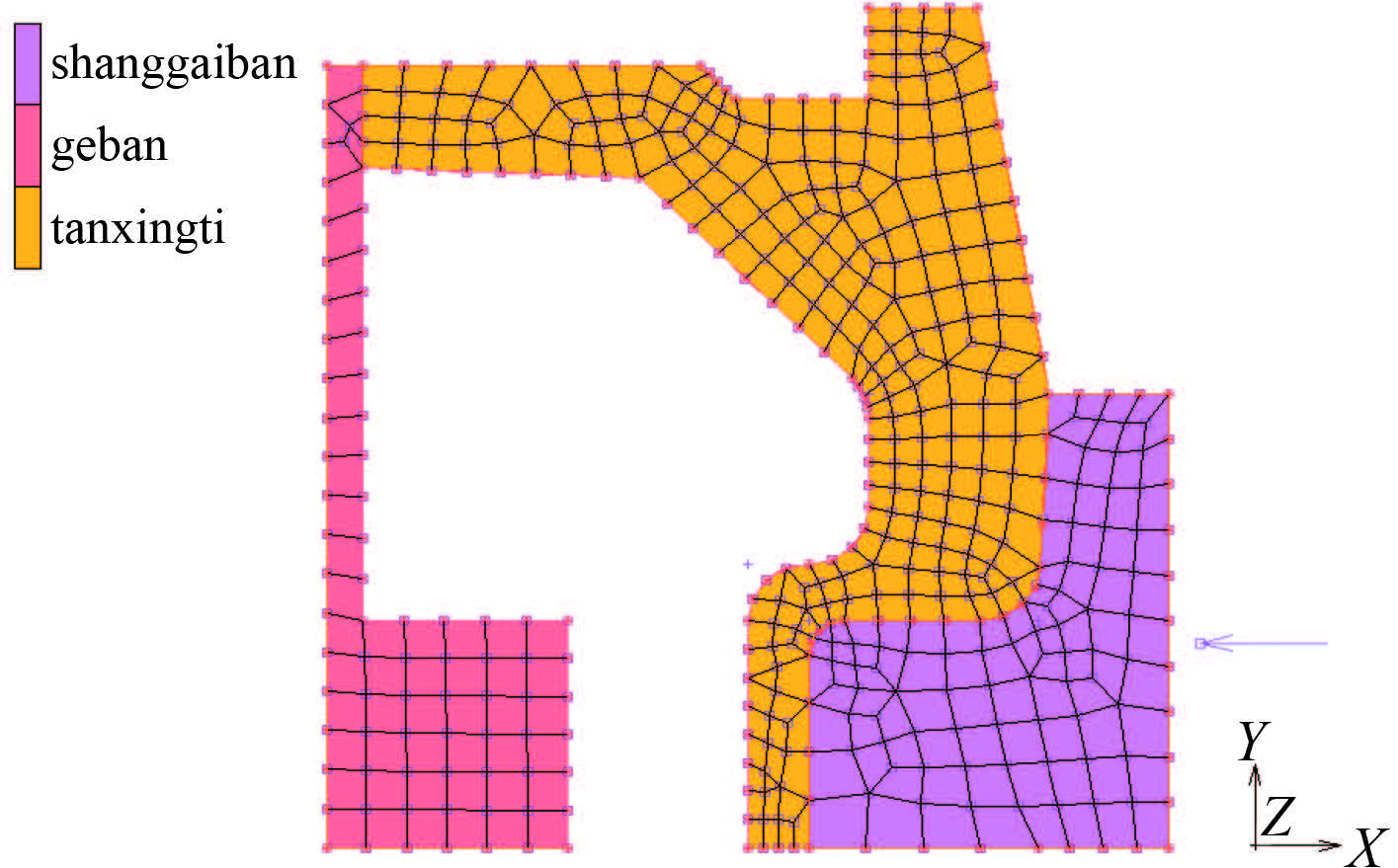图 7 隔振器的有限元模型 Fig. 7 Finite element model of air spring
2.3 有限元计算结果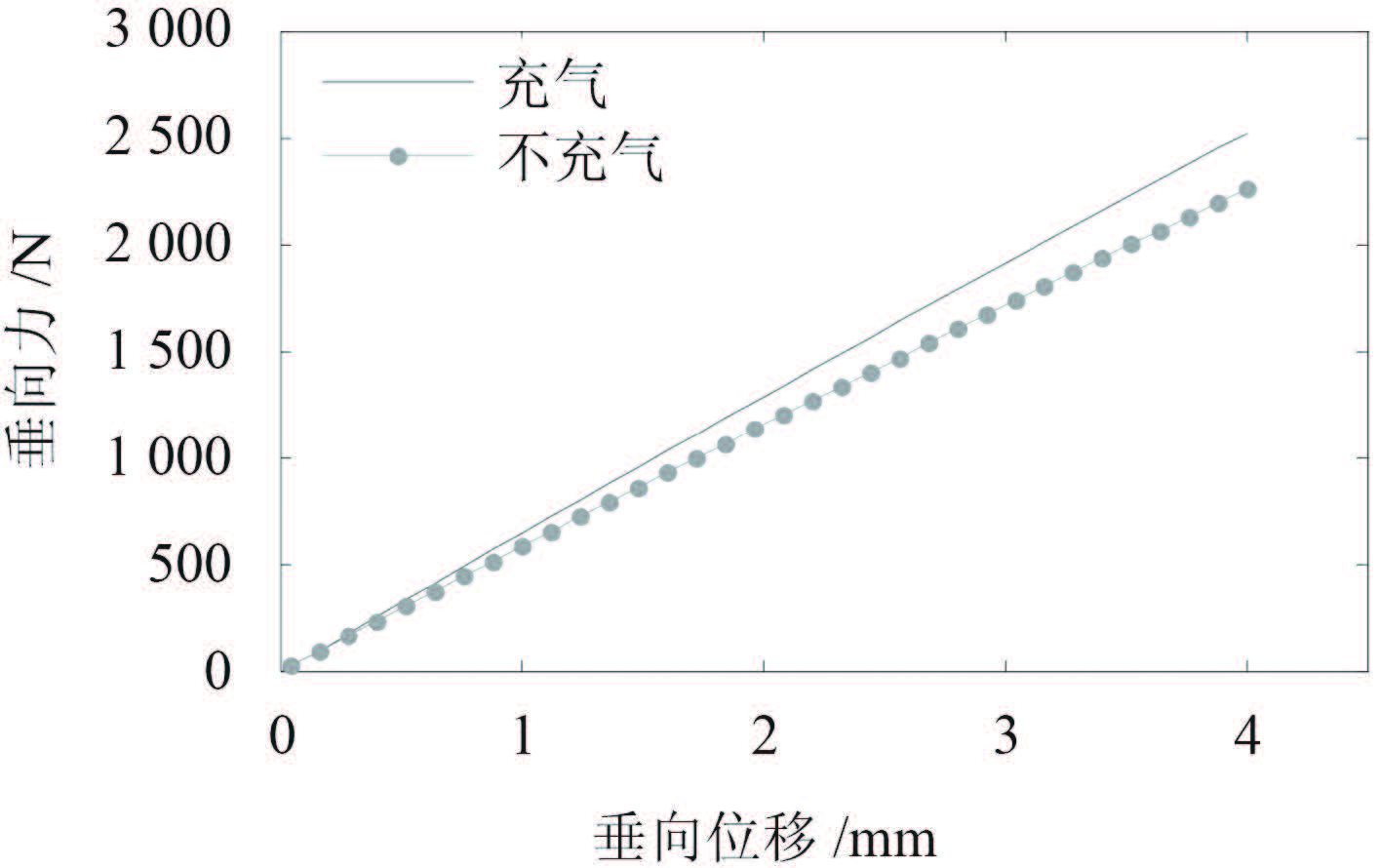图 8 垂向静态特性有限元计算结果 Fig. 8 Vertical static properties of finite element analysis
3 实验验证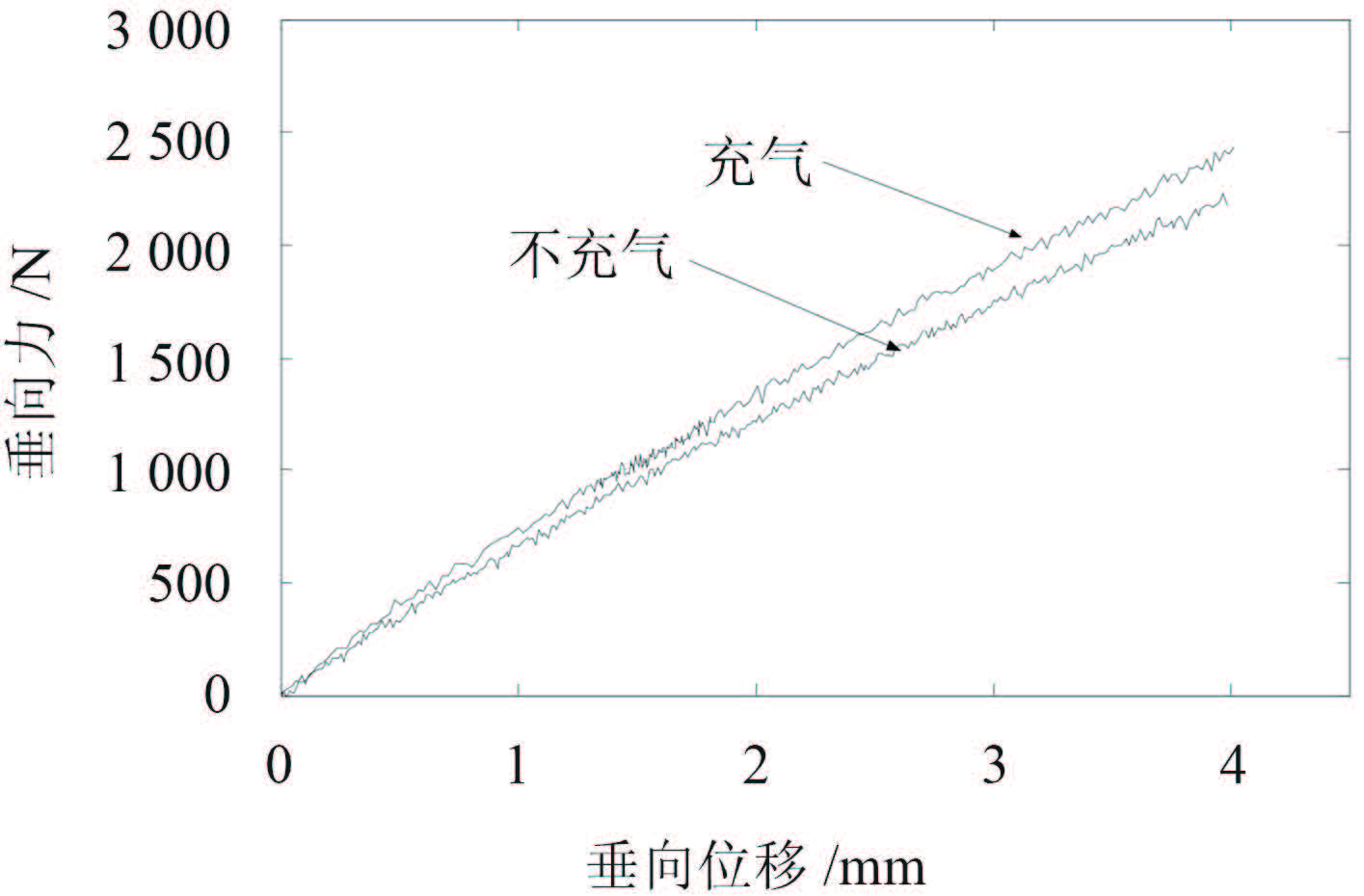图 9 垂向静态特性实验结果 Fig. 9 Vertical static properties of experimental measurement
4 对比分析图 10 不充气时隔振器垂向静态特性 Fig. 10 Vertical static properties of complex air spring in not inflation situation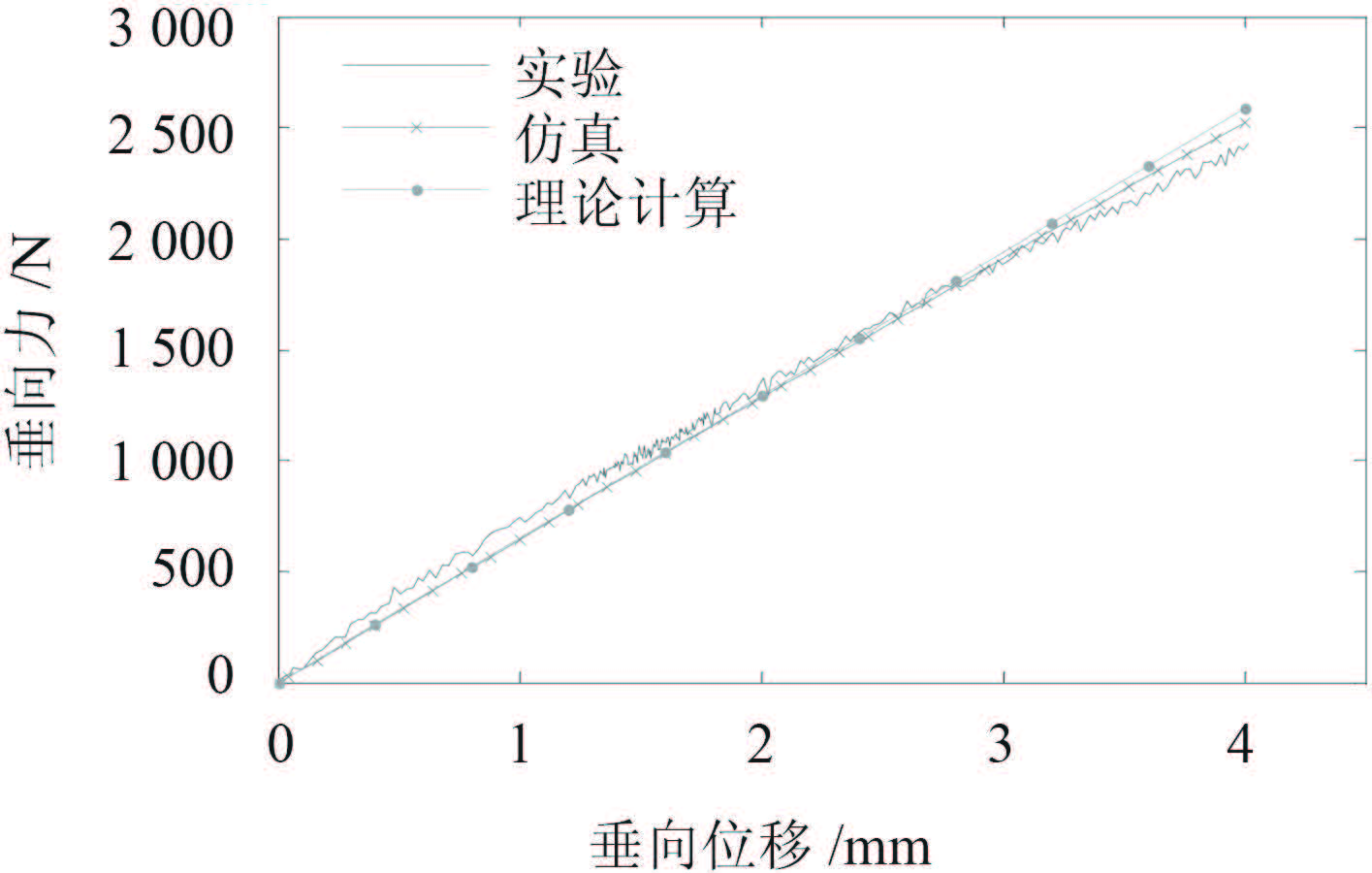图 11 充气时隔振器垂向静态特性 Fig. 11 Vertical static properties of complex air spring in inflation situation表 2 隔振器垂向静刚比较 Tab.2 Comparisonof vertical static properties of air spring

5 结 语

1）轴系横向振动模态频率对轴承刚度、基座刚度存在敏感区，轴系设计时可根据模态频率对刚度的敏感情况适当调整轴承刚度和基座刚度；

2）轴系横向振动存在的部分稳定模态频率，不随轴承刚度、基座刚度变化，若该阶模态频率为振动控制对象，改变轴系支撑刚度难以达到优化模态频率的控制目标；

3）对于 4#轴承，强基座刚度、弱轴承刚度，有利于降低螺旋桨横向激励力通过轴系向 4#轴承的传递；

4）对于舱内油润滑轴承，轴承刚度、基座刚度改变对螺旋桨激励通过轴系向 4#轴承的传递影响较小，轴系横向振动控制设计时可忽略。

  朱石坚, 何琳. 船舶减振降噪技术与工程设计[M]. 北京: 科学出版社, 2002 .  赵应龙, 吕志强, 何琳. JYQN舰用气囊隔振器研究[J]. 舰船科学技术 , 2006, 28 (S2) :89–92. ZHAO Ying-long, LV Zhi-qiang, HE Lin. Study of pneumatic vibration isolator of type JYQN[J]. Ship Science and Technology , 2006, 28 (S2) :89–92.  吴恒亮, 代会军. 橡胶隔振器设计开发研究[J]. 噪声与振动控制 , 2009, 29 (1) :114–116. WU Heng-liang, DAI Hui-jun. Application of finite element analysis in design and development of rubber isolators[J]. Noise and Vibration Control , 2009, 29 (1) :114–116.  师汉民, 黄其柏. 机械振动系统[M].版本. 武汉: 华中科技大学出版社, 2013 .  严济宽. 机械振动隔离技术[M]. 上海: 上海科学技术文献出版社, 1985 .  刘柏峰. 聚氨酯隔振器性能分析与试验研究[D]. 武汉:海军工程大学, 2010.  户原春彦. 防振橡胶及其应用[M]. 北京: 中国铁道出版社, 1982 .  OGDEN R W. Non-linear elastic deformations[M]. Chichester, UK: Ellis Horwood, 1984 .